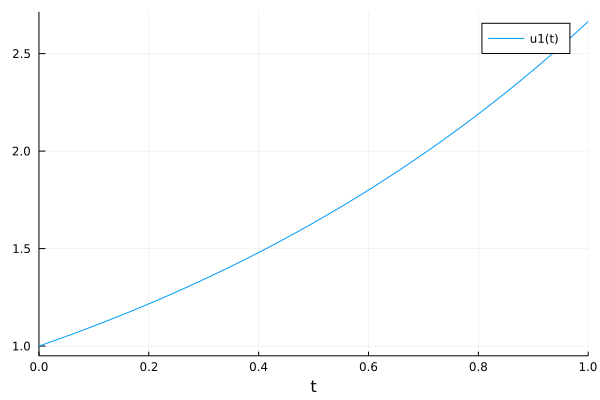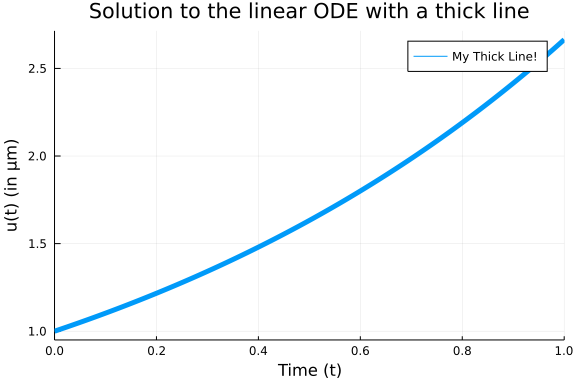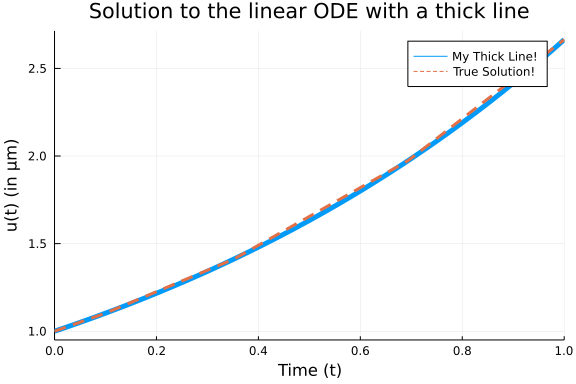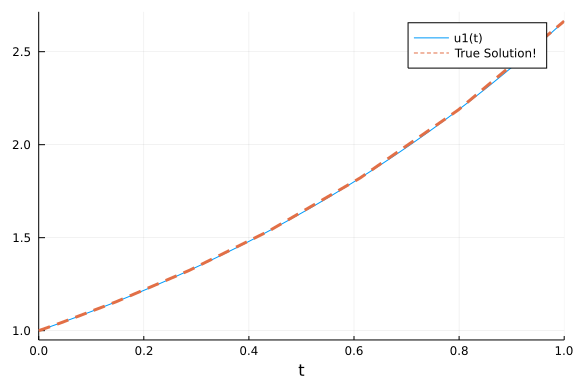# An Intro to DifferentialEquations.jl

## Basic Introduction Via Ordinary Differential Equations

This notebook will get you started with DifferentialEquations.jl by introducing you to the functionality for solving ordinary differential equations (ODEs). The corresponding documentation page is the ODE tutorial. While some of the syntax may be different for other types of equations, the same general principles hold in each case. Our goal is to give a gentle and thorough introduction that highlights these principles in a way that will help you generalize what you have learned.

### Background

If you are new to the study of differential equations, it can be helpful to do a quick background read on the definition of ordinary differential equations. We define an ordinary differential equation as an equation which describes the way that a variable $u$ changes, that is

$u' = f(u,p,t)$

where $p$ are the parameters of the model, $t$ is the time variable, and $f$ is the nonlinear model of how $u$ changes. The initial value problem also includes the information about the starting value:

$u(t_0) = u_0$

Together, if you know the starting value and you know how the value will change with time, then you know what the value will be at any time point in the future. This is the intuitive definition of a differential equation.

### First Model: Exponential Growth

Our first model will be the canonical exponential growth model. This model says that the rate of change is proportional to the current value, and is this:

$u' = au$

where we have a starting value $u(0)=u_0$. Let's say we put 1 dollar into Bitcoin which is increasing at a rate of $98\%$ per year. Then calling now $t=0$ and measuring time in years, our model is:

$u' = 0.98u$

and $u(0) = 1.0$. We encode this into Julia by noticing that, in this setup, we match the general form when

f(u,p,t) = 0.98u

f (generic function with 1 method)


with $u_0 = 1.0$. If we want to solve this model on a time span from t=0.0 to t=1.0, then we define an ODEProblem by specifying this function f, this initial condition u0, and this time span as follows:

using DifferentialEquations
f(u,p,t) = 0.98u
u0 = 1.0
tspan = (0.0,1.0)
prob = ODEProblem(f,u0,tspan)

ODEProblem with uType Float64 and tType Float64. In-place: false
timespan: (0.0, 1.0)
u0: 1.0


To solve our ODEProblem we use the command solve.

sol = solve(prob)

retcode: Success
Interpolation: automatic order switching interpolation
t: 5-element Vector{Float64}:
0.0
0.10042494449239292
0.35218603951893646
0.6934436028208104
1.0
u: 5-element Vector{Float64}:
1.0
1.1034222047865465
1.4121908848175448
1.9730384275622996
2.664456142481451


and that's it: we have succesfully solved our first ODE!

#### Analyzing the Solution

Of course, the solution type is not interesting in and of itself. We want to understand the solution! The documentation page which explains in detail the functions for analyzing the solution is the Solution Handling page. Here we will describe some of the basics. You can plot the solution using the plot recipe provided by Plots.jl:

using Plots; gr()
plot(sol)From the picture we see that the solution is an exponential curve, which matches our intuition. As a plot recipe, we can annotate the result using any of the Plots.jl attributes. For example:

plot(sol,linewidth=5,title="Solution to the linear ODE with a thick line",
xaxis="Time (t)",yaxis="u(t) (in μm)",label="My Thick Line!") # legend=falseUsing the mutating plot! command we can add other pieces to our plot. For this ODE we know that the true solution is $u(t) = u_0 exp(at)$, so let's add some of the true solution to our plot:

plot!(sol.t, t->1.0*exp(0.98t),lw=3,ls=:dash,label="True Solution!")In the previous command I demonstrated sol.t, which grabs the array of time points that the solution was saved at:

sol.t

5-element Vector{Float64}:
0.0
0.10042494449239292
0.35218603951893646
0.6934436028208104
1.0


We can get the array of solution values using sol.u:

sol.u

5-element Vector{Float64}:
1.0
1.1034222047865465
1.4121908848175448
1.9730384275622996
2.664456142481451


sol.u[i] is the value of the solution at time sol.t[i]. We can compute arrays of functions of the solution values using standard comprehensions, like:

[t+u for (u,t) in tuples(sol)]

5-element Vector{Float64}:
1.0
1.2038471492789395
1.7643769243364813
2.66648203038311
3.664456142481451


However, one interesting feature is that, by default, the solution is a continuous function. If we check the print out again:

sol

retcode: Success
Interpolation: automatic order switching interpolation
t: 5-element Vector{Float64}:
0.0
0.10042494449239292
0.35218603951893646
0.6934436028208104
1.0
u: 5-element Vector{Float64}:
1.0
1.1034222047865465
1.4121908848175448
1.9730384275622996
2.664456142481451


you see that it says that the solution has a order changing interpolation. The default algorithm automatically switches between methods in order to handle all types of problems. For non-stiff equations (like the one we are solving), it is a continuous function of 4th order accuracy. We can call the solution as a function of time sol(t). For example, to get the value at t=0.45, we can use the command:

sol(0.45)

1.554261048055312


#### Controlling the Solver

DifferentialEquations.jl has a common set of solver controls among its algorithms which can be found at the Common Solver Options page. We will detail some of the most widely used options.

The most useful options are the tolerances abstol and reltol. These tell the internal adaptive time stepping engine how precise of a solution you want. Generally, reltol is the relative accuracy while abstol is the accuracy when u is near zero. These tolerances are local tolerances and thus are not global guarantees. However, a good rule of thumb is that the total solution accuracy is 1-2 digits less than the relative tolerances. Thus for the defaults abstol=1e-6 and reltol=1e-3, you can expect a global accuracy of about 1-2 digits. If we want to get around 6 digits of accuracy, we can use the commands:

sol = solve(prob,abstol=1e-8,reltol=1e-8)

retcode: Success
Interpolation: automatic order switching interpolation
t: 9-element Vector{Float64}:
0.0
0.04127492324135852
0.14679917846877366
0.28631546412766684
0.4381941361169628
0.6118924302028597
0.7985659100883337
0.9993516479536952
1.0
u: 9-element Vector{Float64}:
1.0
1.0412786454705882
1.1547261252949712
1.3239095703537043
1.5363819257509728
1.8214895157178692
2.1871396448296223
2.662763824115295
2.664456241933517


Now we can see no visible difference against the true solution:

plot(sol)
plot!(sol.t, t->1.0*exp(0.98t),lw=3,ls=:dash,label="True Solution!")Notice that by decreasing the tolerance, the number of steps the solver had to take was 9 instead of the previous 5. There is a trade off between accuracy and speed, and it is up to you to determine what is the right balance for your problem.

Another common option is to use saveat to make the solver save at specific time points. For example, if we want the solution at an even grid of t=0.1k for integers k, we would use the command:

sol = solve(prob,saveat=0.1)

retcode: Success
Interpolation: 1st order linear
t: 11-element Vector{Float64}:
0.0
0.1
0.2
0.3
0.4
0.5
0.6
0.7
0.8
0.9
1.0
u: 11-element Vector{Float64}:
1.0
1.102962785129292
1.2165269512238264
1.341783821227542
1.4799379510586077
1.632316207054161
1.8003833264983584
1.9857565541588758
2.1902158127997695
2.415725742084496
2.664456142481451


Notice that when saveat is used the continuous output variables are no longer saved and thus sol(t), the interpolation, is only first order. We can save at an uneven grid of points by passing a collection of values to saveat. For example:

sol = solve(prob,saveat=[0.2,0.7,0.9])

retcode: Success
Interpolation: 1st order linear
t: 3-element Vector{Float64}:
0.2
0.7
0.9
u: 3-element Vector{Float64}:
1.2165269512238264
1.9857565541588758
2.415725742084496


If we need to reduce the amount of saving, we can also turn off the continuous output directly via dense=false:

sol = solve(prob,dense=false)

retcode: Success
Interpolation: 1st order linear
t: 5-element Vector{Float64}:
0.0
0.10042494449239292
0.35218603951893646
0.6934436028208104
1.0
u: 5-element Vector{Float64}:
1.0
1.1034222047865465
1.4121908848175448
1.9730384275622996
2.664456142481451


and to turn off all intermediate saving we can use save_everystep=false:

sol = solve(prob,save_everystep=false)

retcode: Success
Interpolation: 1st order linear
t: 2-element Vector{Float64}:
0.0
1.0
u: 2-element Vector{Float64}:
1.0
2.664456142481451


If we want to solve and only save the final value, we can even set save_start=false.

sol = solve(prob,save_everystep=false,save_start = false)

retcode: Success
Interpolation: 1st order linear
t: 1-element Vector{Float64}:
1.0
u: 1-element Vector{Float64}:
2.664456142481451


Note that similarly on the other side there is save_end=false.

More advanced saving behaviors, such as saving functionals of the solution, are handled via the SavingCallback in the Callback Library which will be addressed later in the tutorial.

#### Choosing Solver Algorithms

There is no best algorithm for numerically solving a differential equation. When you call solve(prob), DifferentialEquations.jl makes a guess at a good algorithm for your problem, given the properties that you ask for (the tolerances, the saving information, etc.). However, in many cases you may want more direct control. A later notebook will help introduce the various algorithms in DifferentialEquations.jl, but for now let's introduce the syntax.

The most crucial determining factor in choosing a numerical method is the stiffness of the model. Stiffness is roughly characterized by a Jacobian f with large eigenvalues. That's quite mathematical, and we can think of it more intuitively: if you have big numbers in f (like parameters of order 1e5), then it's probably stiff. Or, as the creator of the MATLAB ODE Suite, Lawrence Shampine, likes to define it, if the standard algorithms are slow, then it's stiff. We will go into more depth about diagnosing stiffness in a later tutorial, but for now note that if you believe your model may be stiff, you can hint this to the algorithm chooser via alg_hints = [:stiff].

sol = solve(prob,alg_hints=[:stiff])

retcode: Success
Interpolation: specialized 3rd order "free" stiffness-aware interpolation
t: 8-element Vector{Float64}:
0.0
0.05653299582822294
0.17270731152826024
0.3164602871490142
0.5057500163821153
0.7292241858994543
0.9912975001018789
1.0
u: 8-element Vector{Float64}:
1.0
1.0569657840332976
1.1844199383303913
1.3636037723365293
1.6415399686182572
2.043449143475479
2.6418256160577602
2.6644526430553808


Stiff algorithms have to solve implicit equations and linear systems at each step so they should only be used when required.

If we want to choose an algorithm directly, you can pass the algorithm type after the problem as solve(prob,alg). For example, let's solve this problem using the Tsit5() algorithm, and just for show let's change the relative tolerance to 1e-6 at the same time:

sol = solve(prob,Tsit5(),reltol=1e-6)

retcode: Success
Interpolation: specialized 4th order "free" interpolation
t: 10-element Vector{Float64}:
0.0
0.028970819746309166
0.10049147151547619
0.19458908698515082
0.3071725081673423
0.43945421453622546
0.5883434923759523
0.7524873357619015
0.9293021330536031
1.0
u: 10-element Vector{Float64}:
1.0
1.0287982807225062
1.1034941463604806
1.2100931078233779
1.351248605624241
1.538280340326815
1.7799346012651116
2.090571742234628
2.486102171447025
2.6644562434913377


### Systems of ODEs: The Lorenz Equation

Now let's move to a system of ODEs. The Lorenz equation is the famous "butterfly attractor" that spawned chaos theory. It is defined by the system of ODEs:

\begin{align} \frac{dx}{dt} &= \sigma (y - x)\\ \frac{dy}{dt} &= x (\rho - z) -y\\ \frac{dz}{dt} &= xy - \beta z \end{align}

To define a system of differential equations in DifferentialEquations.jl, we define our f as a vector function with a vector initial condition. Thus, for the vector u = [x,y,z]', we have the derivative function:

function lorenz!(du,u,p,t)
σ,ρ,β = p
du = σ*(u-u)
du = u*(ρ-u) - u
du = u*u - β*u
end

lorenz! (generic function with 1 method)


Notice here we used the in-place format which writes the output to the preallocated vector du. For systems of equations the in-place format is faster. We use the initial condition $u_0 = [1.0,0.0,0.0]$ as follows:

u0 = [1.0,0.0,0.0]

3-element Vector{Float64}:
1.0
0.0
0.0


Lastly, for this model we made use of the parameters p. We need to set this value in the ODEProblem as well. For our model we want to solve using the parameters $\sigma = 10$, $\rho = 28$, and $\beta = 8/3$, and thus we build the parameter collection:

p = (10,28,8/3) # we could also make this an array, or any other type!

(10, 28, 2.6666666666666665)


Now we generate the ODEProblem type. In this case, since we have parameters, we add the parameter values to the end of the constructor call. Let's solve this on a time span of t=0 to t=100:

tspan = (0.0,100.0)
prob = ODEProblem(lorenz!,u0,tspan,p)

ODEProblem with uType Vector{Float64} and tType Float64. In-place: true
timespan: (0.0, 100.0)
u0: 3-element Vector{Float64}:
1.0
0.0
0.0


Now, just as before, we solve the problem:

sol = solve(prob)

retcode: Success
Interpolation: automatic order switching interpolation
t: 1300-element Vector{Float64}:
0.0
3.5678604836301404e-5
0.0003924646531993154
0.003262408518896374
0.009058077168386882
0.01695647153663815
0.027689960628879868
0.041856351821061455
0.06024041060823337
0.08368540639551347
⋮
99.25227436435598
99.34990050231407
99.47329750836677
99.56888278883171
99.68067056500875
99.7698930548574
99.86396908592394
99.9520070464327
100.0
u: 1300-element Vector{Vector{Float64}}:
[1.0, 0.0, 0.0]
[0.9996434557625105, 0.0009988049817849058, 1.781434788799189e-8]
[0.9961045497425811, 0.010965399721242457, 2.1469553658389193e-6]
[0.9693591566959717, 0.089770627357676, 0.0001438019004555601]
[0.9242043510496474, 0.24228916412927515, 0.0010461626692665619]
[0.8800455755115648, 0.43873651254178225, 0.003424260317913913]
[0.8483309815012585, 0.6915629798778471, 0.008487625758932924]
[0.8495036692770451, 1.0145426674126548, 0.018212090760571238]
[0.9139069519040545, 1.4425599553295452, 0.036693820689070726]
[1.088863767524423, 2.052326420986981, 0.07402572431671739]
⋮
[5.791198832787258, 3.8198742723079415, 26.666685868569655]
[4.959577668148714, 5.1627464679185815, 22.495413403699086]
[6.653100232410356, 9.122429705812463, 20.41123180056622]
[9.593094878342031, 12.952212134722183, 23.567760855989487]
[11.932162424256417, 11.761476358727544, 31.642393421283604]
[9.907977700774161, 6.112102601076882, 32.83064925680822]
[6.4119417247899015, 3.408711533396652, 28.483033292250997]
[4.734282403550539, 3.913681157796059, 24.002168300285124]
[4.596259899368738, 4.819051128437629, 22.02318896633189]


The same solution handling features apply to this case. Thus sol.t stores the time points and sol.u is an array storing the solution at the corresponding time points.

However, there are a few extra features which are good to know when dealing with systems of equations. First of all, sol also acts like an array. sol[i] returns the solution at the ith time point.

sol.t,sol

(0.08368540639551347, [1.088863767524423, 2.052326420986981, 0.074025724316
71739])


Additionally, the solution acts like a matrix where sol[j,i] is the value of the jth variable at time i:

sol[2,10]

2.052326420986981


We can get a real matrix by performing a conversion:

A = Array(sol)

3×1300 Matrix{Float64}:
1.0  0.999643     0.996105    0.969359     …   6.41194   4.73428   4.59626
0.0  0.000998805  0.0109654   0.0897706        3.40871   3.91368   4.81905
0.0  1.78143e-8   2.14696e-6  0.000143802     28.483    24.0022   22.0232


This is the same as sol, i.e. sol[i,j] = A[i,j], but now it's a true matrix. Plotting will by default show the time series for each variable:

plot(sol)